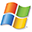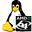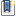ATOMS : Condition Number details

# Condition Number

Evaluates the condition number of functions.
Details
Version
0.2.2
Author
Michael Baudin
Owner Organization
DIGITEO
Maintainers
John Gliksberg
Michael Baudin
Category
Dependency
Creation Date
September 7, 2015
Source created on
Scilab 6.0.x
Binaries available on
Scilab 6.0.x:Windows 64-bitWindows 32-bitLinux 64-bitLinux 32-bitMacOSX
Install command
`--> atomsInstall("condnb")`
Report a bugDescription
```            Purpose
----

These functions provides the condition number of various elementary functions.
Their goal is to evaluate the potential numerical difficulty of evaluating
numerically a function at a given point.
We may use this module while testing a given function.

The toolbox is based on macros.

To get an overview of the functions in this module, use :

help condnb_overview

This module uses the apifun module.

Features
--------

The following is a list of the current functions :
* condnb_condnum : Computes the empirical condition number of the function f at
point x.
* condnb_acoscond : Computes the condition number of the acos function.
* condnb_asincond : Computes the condition number of the asin function.
* condnb_atancond : Computes the condition number of the atan function.
* condnb_coscond : Computes the condition number of the cos function.
* condnb_erfcond : Computes the condition number of the erf function.
* condnb_erfinvcond : Computes the condition number of the erfinv function.
* condnb_expcond : Computes the condition number of the exp function.
* condnb_logcond : Computes the condition number of the log function.
* condnb_plotcond : Plots the condition number of a function.
* condnb_powcond : Computes the condition number of the pow function.
* condnb_sincond : Computes the condition number of the sin function.
* condnb_sqrtcond : Computes the condition number of the square root function.
* condnb_sumcond : Computes the condition number of the sum function.
* condnb_tancond : Computes the condition number of the tan function.

Forge
-----

http://forge.scilab.org/index.php/p/condnb/
```Files (2)Source code archive
```
```OS-independent binary for Scilab 6.0.x
```Binary version
Automatically generated by the ATOMS compilation chain

```News (0)Comments (0)Leave a comment
You must register and log in before leaving a comment.Email notifications
Send me email when this toolbox has changes, new files or a new release.